Browse by Topic
Related Topics

# Adventures into the Mathematical Forest of Fractal Trees

Without doubt, the golden ratio is nowadays considered the most mysterious, magical, and fascinating number that exists: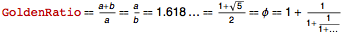. As we will see in this post, this number still has many interesting properties that can be investigated, some even dating back to the works of the ancient Greeks Pythagoras and Euclid, the Italian mathematician Leonardo of Pisa, and the Renaissance astronomer Johannes Kepler. Though it might sound strange, I will unveil new geometric objects associated with the golden ratio, which are the objects that illuminated my way when I attempted to map an unknown region of the Mathematical Forest.

The following findings aren’t a mere accident; I’ve been working hard to grasp a glimpse of new knowledge since high school. After seeing Hans Walser‘s drawings of golden fractal trees in 2007, I was convinced that there was still space for exploration and new discoveries. Though I had to wait quite a while, I finally found the right tools: Mathematica, combined with Theo Gray‘s “Tree Bender” Demonstration. After gathering some intuition and a rudimentary knowledge of the Wolfram Language, I encountered my first insights. For example, here is one of the first self-contacting golden trees that I discovered when I created my own version of “Tree Bender” in order to explore ternary trees (trees with three branches per node):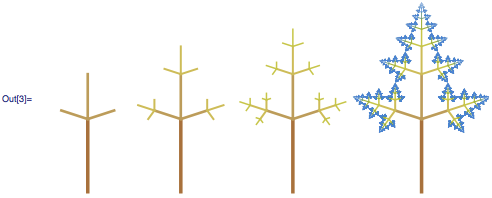This is a self-similar tree, a tree that can be regarded as a substitution system where a branching rule is applied recursively. I call “golden trees” trees with branches scaling according to a multiple of GoldenRatio = ϕ. For this particular tree, the scaling factor is ϕ-1 for the middle branches and ϕ-2 for the symmetrical pairs. The angle of the symmetrical pairs is 72º from the middle branches. As it has neither branch-crossing nor disconnected leaves, it is said to be “self-contacting.” Let’s look at some of its properties more closely: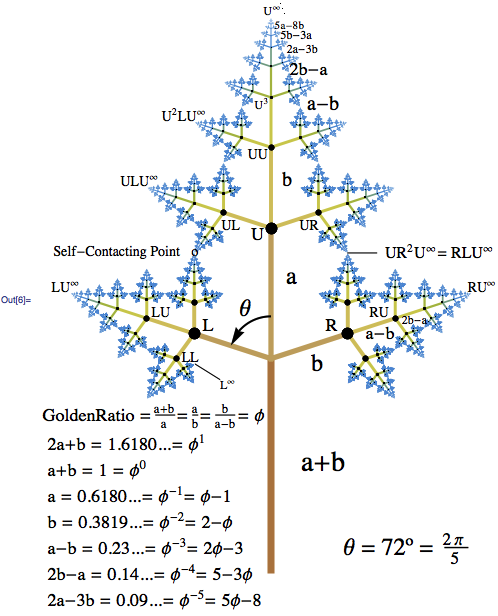Following the notation introduced by Benoit Mandelbrot and Michael Frame for binary trees, I added a third letter U to map all the branches of a symmetric ternary tree. The letter L is for branches turning left, R for branches turning right, and U for branches going up. So a string of letters is an address path that maps to a unique branch. When the address is infinite, we call the limiting branch a tip or an attracting fixed point. For example, the infinite address UUU... = U describes the tip at the top of the tree: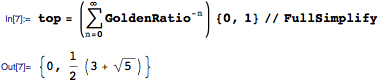So the height of this tree is: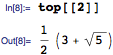And its width is the distance between tip LU and RU: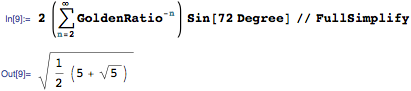It’s also interesting to notice that the length of the successive branches can be represented using the Fibonacci numbers. See expressions at the bottom-left of the preceding diagram: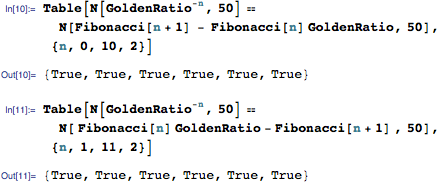Finally, to prove that this tree is self-contacting, we just need to show that two different leaves (or tips) connect in one of the two main tip-to-tip self-contacting points labeled in the diagram below: UR2 U = RLU and its mirror-symmetric self-contacting point (left side). If that’s the case, then the self-similarity of the tree implies that there is no tip disconnected, that is, the tip set is connected. Take any tip, cut the subset that looks like the whole tree, scale and rotate by the right amount, and you will have one of the two main self-contacting points: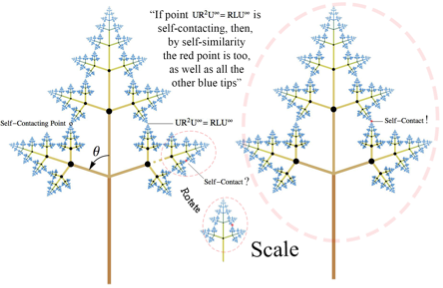For tip UR2U, the coordinates are determined by summing up the following terms: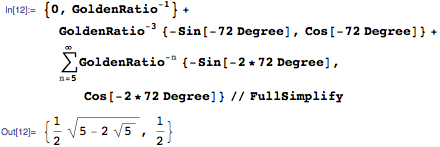And for tip RLU, the coordinates are determined by: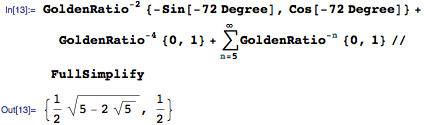Because these two coordinates are identical, we can confirm it’s a self-contacting fractal tree.

Another fascinating aspect of this golden tree is that it creates this nice pattern with 5-fold rotational symmetry when four copies of it are added around the base of the trunk: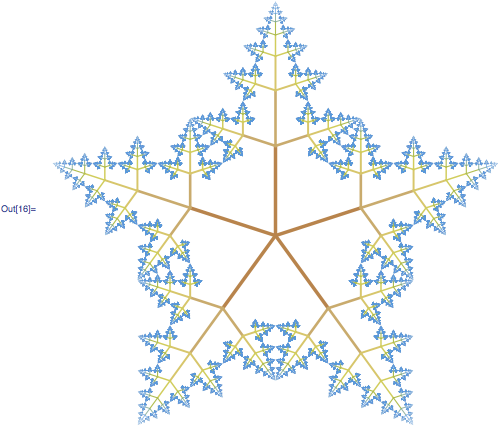Or this other pattern when the copies are placed around the top of the tree: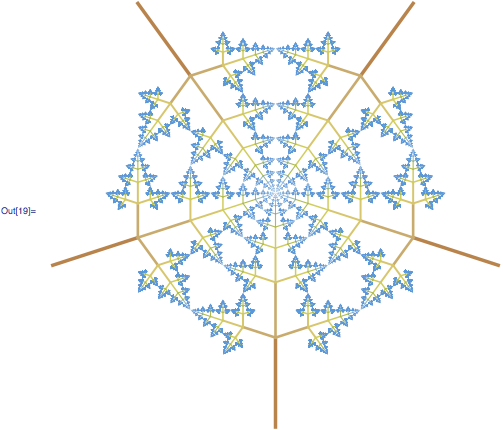I discovered a second golden ternary tree on the same day as the previous one. It is a tree with the middle branch going down D and the left L and right R branches turning at angles of 108º from the linear extension of the trunk: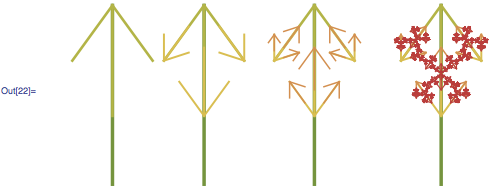This time, when copies of the tree are assembled around the bottom of the trunk, a pattern with 10-fold rotational symmetry emerges: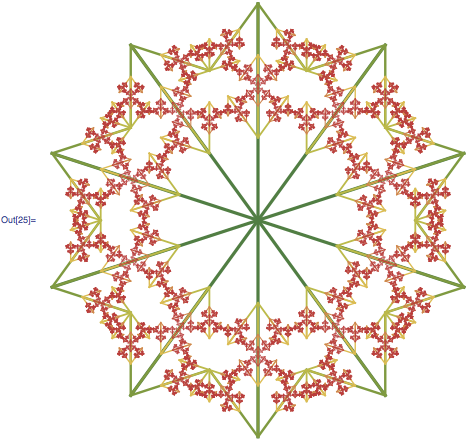Now let me show you the very first golden tree I discovered back in 2011: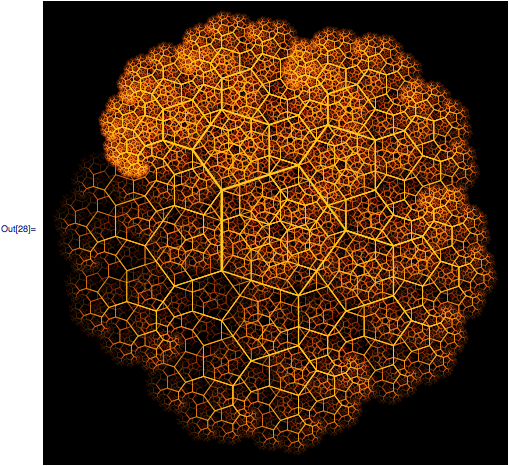This binary tree is asymmetric. The branches turning left are set to scale by a factor equal to GoldenRatio-1 in angles of 36º from the linear extension of its mother branch. And the branches turning right are set to form closed loops of regular pentagons. Its first four generations are depicted below: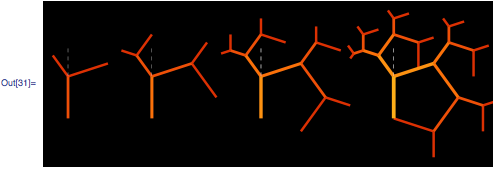When this asymmetrical tree is set to be mirror-symmetric with respect to the linear extension of the trunk, the right R branch as a mirrored copy of the left L branch, the following tree appears: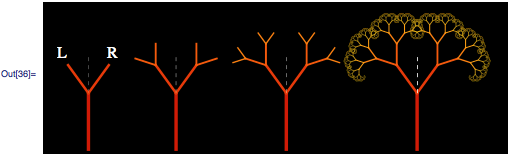This tree can be assembled forming a connected pattern with 5-fold symmetry: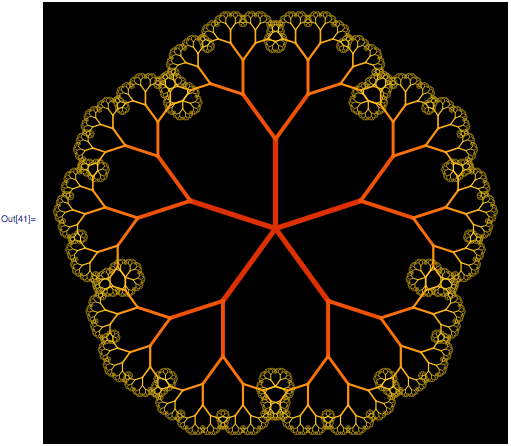The real magic came when I folded this tree, as shown in this animated GIF exported from Mathematica: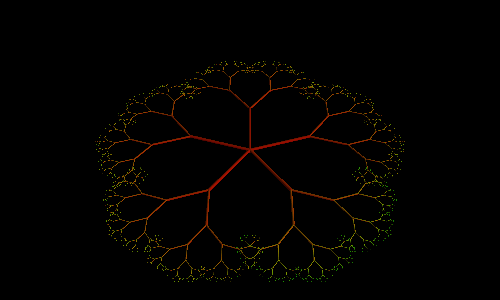When the branches are completely folded up, the tree’s tip set draws a golden Koch snowflake. The GoldenRatio makes the branches line up, forming golden triangles and golden gnomons at all levels of magnification!A family of self-contacting binary trees appeared when the pair of mother branches was constrained to move in a straight horizontal line, as I showed in the Wolfram Community post “Doodling in Mathematica: DRAGON TREES“: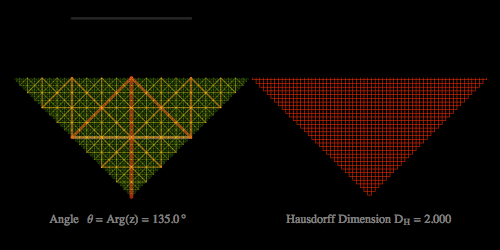Next I explored similar trees with more than two branches per node. Robert Fathauer’s Compendium of Fractal Tilings inspired me to figure out a way to map all these possible Koch-like trees that I was finding, in a single diagram. The diagram was presented last year at the Bridges conference (the paper is available here, figure 4). These direct observations allowed me to take a step forward and generalize the symmetric self-contacting binary fractal trees studied by Benoit Mandelbrot and Michael Frame , Tara Taylor  , Dušan Pagon , and Stephen Wolfram . After tedious work figuring out how the address paths of the branches leading to tip-to-tip self-contact look when the angle θ changes, and the number of branches per node increases, Mathematica provided me the only nine types of equations that determine the self-contacting ratio rsc for the n-ary symmetric fractal trees. I won’t enter into details here—you can explore down self-contacting trees below. If you are interested, I invite you to read this post I wrote in Wolfram Community. The post introduces the results published in Symmetry (Volume 24, Numbers 1–4, pages 320–338, 2013).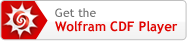My adventures didn’t stop there. Last summer, during my first week at the Wolfram Science Summer School, I had the good fortune to discover the five three-dimensional self-contacting infinite skew trees that scale with the golden ratio (see the Koch-like tree that appears at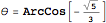below). That was a very exiting moment for me.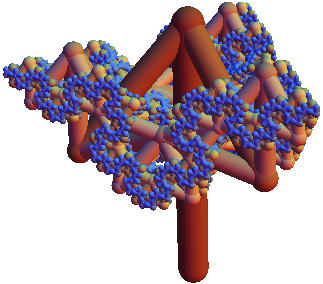When I arrived at the Wolfram Science Summer School, I already had a vague idea of what my project would be about. I wanted to generalize the equations I had found for two-dimensional fractal trees into the 3D space. I had attempted this before, but I had had several issues applying rotations, and I was only able to generate a small collection of highly symmetric trees, like this tetrahedron tree that I 3D-printed just before joining the Summer School. A Demonstration called “Tree Branching in 4D” by Todd Rowland, Academic Director of the Summer School, marked the guidelines for my project, and my mentor, Vitaliy Kaurov, was really optimistic about my proposal.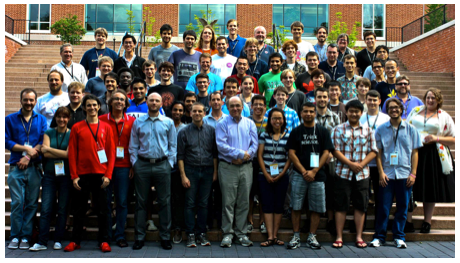During my first conversation with Stephen Wolfram, everyone agreed I should keep my feet in the Mathematical Forest I was coming from, and see if I could climb to a higher dimension. During my first week, I looked for references to three-dimensional fractal trees, and I found the paper “Symmetric Fractal Trees in Three Dimensions,” by Frongillo et al., and Paul Nylander’s example for generating a ternary fractal tree in 3D. From there, I quickly tried to reproduce and extend the results of that paper, based on my intuition and knowledge gained while studying 2D trees under the supervision of Susanne Krömker, in Heidelberg. The final results were astonishing, and I am still amazed how fast all these equations were obtained in three weeks. Certainly, the atmosphere there was the best one could have for carrying out such a project.

“Bottomless wonders spring from simple rules, repeated without end.”

Benoit B. Mandelbrot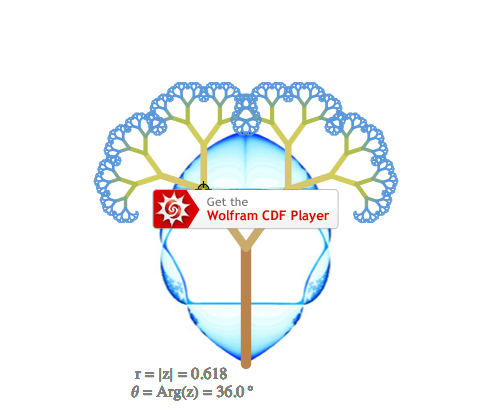(The Manipulate embedded above allows you to explore the forest of symmetric binary fractal trees. The map is the Mandelbrot set for symmetric binary trees. Here, the Julia sets associated with this map are the tip sets of the trees. The map, discovered by Michael Barnsley, has some common properties with the pinhole maps discovered by Stephen Wolfram. The imaginary axis is set to be vertical here in order to have the trees looking upward. The blank interior region is where all the disconnected trees live.)